Slide BasesProduct Name : Slide Bases

Description :

● Posts and Holders components to optical tables
● Depends on space limitation, Provides more flexibility selection in positioning posts and holders.
● Accepts M6 (1/4-20) threaded posts and holders or optomechanics.
Specification

Miniature Slide Base

Model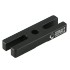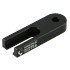Metric  52SDB-1(M) 52SDB-5(M)
English
Slot 2 1
Mounting Hole 1 1
Axis X X
Dimensions W=19mm
L=60mm
H=7.5mm
W=19mm
L=59.5mm
H=7.5mm

Slide Base W=25mm

Model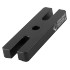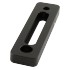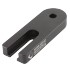Metric  02SDB-1(M) 02SDB-3(M) 02SDB-4M 02SDB-5(M)
English  02SDB-4
Slot 2 1 2 1
Mounting Hole 1 2 1 1
Axis X 360° rotation X X
Dimensions L=25mm
L=76.2mm
H=9.5mm
L=25mm
L=100mm
H=9.5mm
L=25mm
L=75mm
H=9.5mm
L=25mm
L=70mm
H=9.5mm

Slide Base W=50mm

Model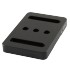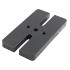Metric  02SDB-2M 02SDB-6M
English  02SDB-2 02SDB-6
Slot 2 2
Mounting Hole 3 3
Axis X X
Dimensions W=50mm
L=75mm
H=9.5mm
W=51mm
L=96mm
H=9.5mm

Multi Axises Slide Base

Model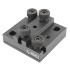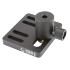Metric  02SDB-7M 02SDB-8M
English  02SDB-7 02SDB-8
Slot 0 5
Mounting Hole 5 0
Axis XY XYθ
Dimensions W=75mm
L=75mm
H=18.8mm
W=70mm
L=76.2mm
H=9.5mm
Model Description Price (USD)
Q'ty
52SDB-1(M) Miniature Slide Base, W=19mm, L=60mm, H=7.5mm, Common Type 6.7
52SDB-5(M) Miniature Slide Base, W=19mm, L=59.5mm, H=7.5mm, Common Type 9
02SDB-1(M) Slide Base, W=25mm L=76.2mm, H=9.5mm, Common Type 5
02SDB-1(M)-PK Slide Base, W=25mm L=76.2mm, H=9.5mm, Common Type, 5 Pack 23.75
02SDB-2M Slide Base, W=50mm, L=75mm, H=9.5mm, Metric Type 6.5
02SDB-3(M) Slide Base, W=25mm L=100mm, H=9.5mm, Common Type 13
02SDB-4M Slide Base, W=25mm L=75mm, H=9.5mm, Metric Type 5.2
02SDB-5(M) Slide Base, W=25mm, L=70mm, H=9.5mm, Common Type 9.5
02SDB-5(M)-PK Slide Base, W=25mm, L=70mm, H=9.5mm, Common Type, 5 Pack 46
02SDB-6M Slide Base, W=51mm, L=96mm, H=9.5mm, Metric Type 15
02SDB-7M Slide Base, W=75mm, L=75mm, H=18.8mm, Metric Type 67
02SDB-8M Slide Base, W=70mm, L=76.2mm, H=9.5mm, Metric Type 60
02SDB-2 Slide Base, W=50mm, L=75mm, H=9.5mm, English Type 6.5
02SDB-4 Slide Base, W=25mm L=75mm, H=9.5mm, English Type 5.2
02SDB-6 Slide Base, W=51, L=96mm, H=9.5mm, English Type 15
02SDB-7 Slide Base, W=75mm, L=75mm, H=18.8mm, English Type 67
02SDB-8 Slide Base, W=70mm, L=76.2mm, H=9.5mm, English Type 60